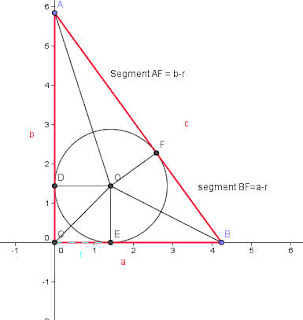## Monday, 21 September 2009

### The Hypotenuse of a Right TriangleIn my last blog I challenged the student to find the length of the diameter of a circle inscribed in a right triangle in terms of the three sides, a, b, and c.
Students often let the "Right Angle" divert them into trying to find something to do with the Pythagorean Theorem, but as I shall show, that is really just putting the horse before the cart. In this case, all they need to see is that the two segments from each vertex are both tangent to the circle, and apply a pretty well known property of tangents from a point.

Using this idea, it seems clear from the image above that Segments AD and AF are congruent, and by looking at the vertical leg of the triangle, we see that both of these are of length b-r. In the same way the segments BE and BF are also congruent, and they both have a length of a-r... but AF + BF = c, so c=a-r+b-r, or c= a+b-2r...

Now just add 2r and subtract c from both sides and we get 2r (the diameter of the circle) = a+b-c.

Of course you could have started out with a look at the area. Since the triangle is made up of three smaller triangles, COB, BOA, and AOC. Each of these areas is 1/2 the product of a side of the triangle and the radius of the circle. So A= 1/2 ar+ 1/2 br+ 1/2 cr which simplifies to A= 1/2 (a+b+c)r ... now expressing the area A as 1/2 ab, we have ab=(a+b+c)r and dividing by the perimeter, we get $\frac{ab}{a+b+c} =r$ . We need only to double that to get the diameter, D=$\frac{2ab}{a+b+c}$.

If we put those together, we get a proof of the Pythagorean theorem... Since the Diameter is equal to both a+b-c and $\frac{2ab}{a+b+c}$ then the two expressions must be equal to each other... so .....

We begin with $\frac{2ab}{a+b+c} =a+b-c$ we multiply through both sides by (a+b+c) to get 2ab= (a+b-c)(a+b+c). Think of this last as [(a+b)+c][(a+b)-c] and so we get 2ab= (a+b)2 -c2.
Now we square the binomial (a+b) to get 2ab= a2+ 2ab+ b2 - c2.

Subtract 2ab from both sides, and we get a2+b2-c2=0... or more simply a2+b2=c2

I love it when stuff like that happens... I should point out for those who couldn't find it, that the difference in the two equilateral triangles formed in the Napoleon Theorem, will always equal the area of the original triangle.